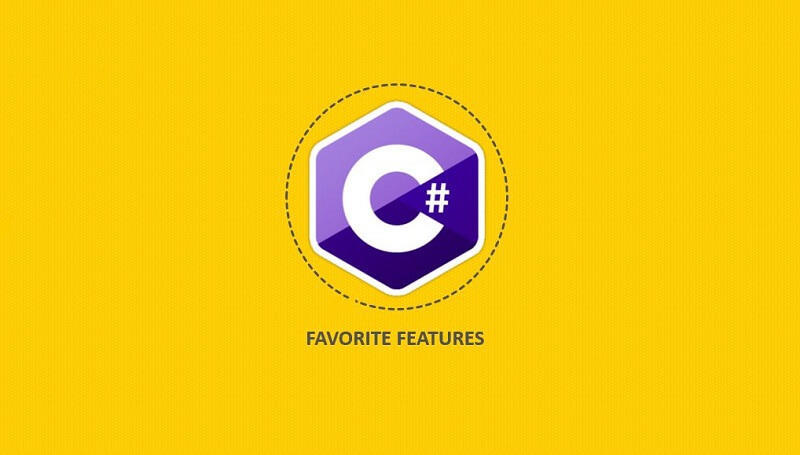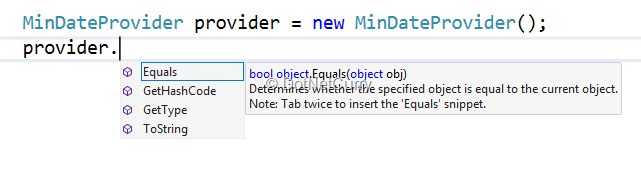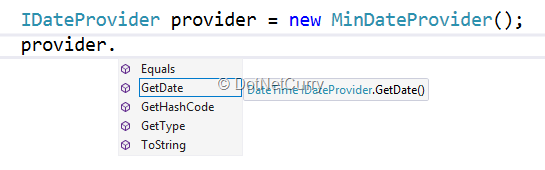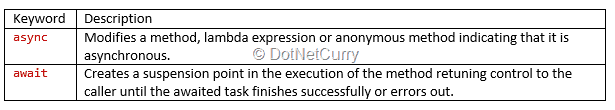#[搬运] C# 这些年来受欢迎的特性

0
0
01. 云栖社区>
2. 博客>
3. 正文

## 原文地址:http://www.dotnetcurry.com/csharp/1411/csharp-favorite-features# C# 1.0

C＃1.0 (ISO-1) 确实算是语言，却没有什么令人兴奋的，缺少许多开发人员喜欢的特性。仔细一想，我能说得出喜欢的只有一个特别的特性 - 隐式和显式接口实现

``````public interface IDateProvider
{
DateTime GetDate();
}``````

``````public class DefaultDateProvider : IDateProvider
{
public DateTime GetDate()
{
return DateTime.Now;
}
}``````

``````public class MinDateProvider : IDateProvider
{
DateTime IDateProvider.GetDate()
{
return DateTime.MinValue;
}
}``````Jeffery Richter 在他 CLR via C# 一书中提醒了我们显式的接口实现两个主要问题是值类型实例在投射到一个接口和明确实现的方法时将被装箱，同时不能被派生类调用。

# C# 2.0

• 匿名方法
• 协变和逆变
• 泛型
• 迭代器
• 可空类型
• 部分类型

``````public class DataBag
{
{
// omitted for brevity...
}
}``````

``````public class DataBag
{
{
// omitted for brevity...
}
}``````

``````public class DataBag where T : struct { /* T 值类型 */ }
public class DataBag where T : class { /* T 类、接口、委托、数组 */ }
public class DataBag where T : new() { /* T 有无参构造函数 */ }
public class DataBag where T : IPerson { /* T 继承 IPerson */ }
public class DataBag where T : BaseClass { /* T 派生自 BaseClass */ }
public class DataBag where T : U { /* T 继承 U, U 也是一个泛型参数 */ }``````

``````public class DataBag where T : class
{
{
// omitted for brevity...
}
}``````

# C# 3.0

• 匿名类型
• 自动实现的属性
• 表达树
• 扩展方法
• Lambda表达
• 查询表达式

``````public class WeatherData
{
public DateTime TimeStampUtc { get; set; }
public decimal Temperature { get; set; }
}

private IEnumerable GetWeatherByZipCode(string zipCode) { /* ... */ }``````

``````private static decimal CalculateAverageTemperature(
IEnumerable weather,
DateTime startUtc,
DateTime endUtc)
{
var sumTemp = 0m;
var total = 0;
foreach (var weatherData in weather)
{
if (weatherData.TimeStampUtc > startUtc &&
weatherData.TimeStampUtc < endUtc)
{
++ total;
sumTemp += weatherData.Temperature;
}
}
return sumTemp / total;
}``````

``````private static decimal CalculateAverageTempatureLambda(
IEnumerable weather,
DateTime startUtc,
DateTime endUtc)
{
return weather.Where(w => w.TimeStampUtc > startUtc &&
w.TimeStampUtc  w.Temperature)
.Average();
}``````

# C# 4.0

• 动态绑定
• 嵌入式互操作类型
• 泛型中的协变和逆变
• 命名/可选参数

``````public class Repository
{
public DataTable GetData(
string storedProcedure,
DateTime start = default(DateTime),
DateTime? end = null,
int? rows = 50,
int? offSet = null)
{
// omitted for brevity...
}
}``````

``````var repo = new Repository();
var sales = repo.GetData("sp_GetHistoricalSales");``````

``````var repo = new Repository();
var sales = repo.GetData("sp_GetHistoricalSales", rows: 100);``````

# C# 5.0

• 异步/等待
• 调用方信息``````class IOBoundAsyncExample
{
// Yes, this is the internet Chuck Norris Database of jokes!
private const string Url = "http://api.icndb.com/jokes/random?limitTo=[nerdy]";

{
using (var client = new HttpClient())
{
var response = await client.GetStringAsync(Url);
var result = JsonConvert.DeserializeObject(response);

return result.Value.Joke;
}
}
}
public class Result
{
[JsonProperty("type")] public string Type { get; set; }
[JsonProperty("value")] public Value Value { get; set; }
}

public class Value
{
[JsonProperty("id")] public int Id { get; set; }
[JsonProperty("joke")] public string Joke { get; set; }
}
``````

# C# 6.0

C# 6.0 有很多很不错的改进，很难选择我最喜欢的特性。

• 字典初始化
• 异常过滤器
• 表达式体成员
• nameof 操作符
• 空合并运算符
• 属性初始化
• 静态引用
• 字符串插值

``````class Person
{
public string FirstName { get; set; }
public string LastName { get; set; }

public override string ToString()
=> string.Format("{0} {1}", FirstName);
}``````

``````class Person
{
public string FirstName { get; set; } = "David";
public string LastName { get; set; } = "Pine";
public DateTime DateOfBirth { get; set; } = new DateTime(1984, 7, 7);

public override string ToString()
=> \$"{FirstName} {LastName} (Born {DateOfBirth:MMMM dd, yyyy})";
}``````

• David Pine (Born July 7, 1984)

# C# 7.0

• 表达式体成员
• 局部方法
• Out 变量
• 模式匹配
• 局部引用和引用返回
• 元组和解构

``````public boolean TryParse(string value, out DateTime date)
{
// omitted for brevity...
}
``````

``````if (DateTime.TryParse(someDateString, out var date))
{
// date is now the parsed value
}
else
{
// date is DateTime.MinValue, the default value
}``````

``````if (DateTime.TryParse(someDateString, out var date))
{
// date is now the parsed value
}
else
{
// date is DateTime.MinValue, the default value
}``````

``````public static class StringExtensions
{
private delegate bool TryParseDelegate(string s, out T result);

private static T To(string value, TryParseDelegate parse)
=> parse(value, out T result) ? result : default;

public static int ToInt32(this string value)
=> To(value, int.TryParse);

public static DateTime ToDateTime(this string value)
=> To(value, DateTime.TryParse);

public static TimeSpan ToTimeSpan(this string value)
=> To(value, TimeSpan.TryParse);
}``````

``````ublic class Program
{
public static void Main(string[] args)
{
var str =
string.Join(
"",
new[] { "James", "Bond", " +7 " }.Select(s => s.ToInt32()));

Console.WriteLine(str); // prints "007"
}
}``````

+ 关注